TI Tips
This document gives some tips on how to use the TI-85 to do numerical calculations, visualize mathematical relations using graphs and how to write simple programs. It covers topics from basic definitions to programming design techniques.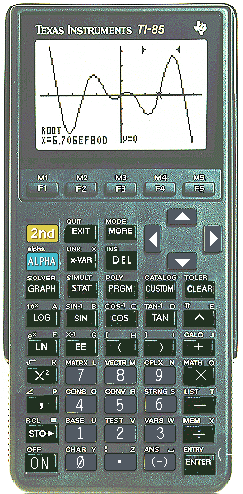It is assumed you have access to a TI-85 calculator and are acquainted with the user's manual (such as it is.)   Much of what's here can be applied a TI-8X or TI-92 as well, with minor changes in syntax.  Be encouraged to experiment for yourself with programming concepts as they are introduced.

We start with some basic stuff you may know already, though perhaps the perspective will be new.  The essentials of variables, graphs, input/output operations and program control mechanisms are introduced - in the context of what I hope are some interesting mathematical applications.  The level of mathematical sophistication varies from basic algebra to sophomore level calculus.

We finish with some tips about program design you can use to start building a kit bag of scripts for accomplishing common programming tasks using the data structures and input/output features available to the TI 85.

I'll be adding to this document occassionally, so come back now and then to see what's new!

I would like to credit the folks at TI (http://www.ti.com/calc/docs/arch.htm) for helping me learn about the '85.

Variables
On the TI-8X and other computers, variables are realized as places in memory in which you can store a numerical value, a group of letters, symbols, lists, arrays, and many other sorts of data. A variable name on the '85 can consist of up to eight numbers and letters, but the first character must be a letter.  To store a value or string of characters in a variable, the syntax:
<value> <variablename> (for a value) or
"words" <variablename> (for words)
To change the value of a variable, just use the same method to store a new value in place of the present value. To recall a variable's value, simply type in the variable's name and press [ENTER].

To see a complete list of variables currently in use on you calculator, press
[2nd]  [F1].  You should get something similar to: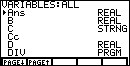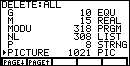On the left is a listing of variables given alphabetically (lower case later.)  On the right is the variable's data type. Use the down arrow to scroll through the list.  To delete a variable, hit [2nd] [+] to bring up the memory menu, and then [F2] [F1] to see a list of all variables, together with their size and type (2nd illustration above.)   Scroll down to the one you want to get rid of and press [ENTER].  Poof, it's gone!
Variable Data Types and Some Associated Operations
Mostly for purposes of memory allocation and to define legal operations, a variable is given a data type (listed in the right hand column of the screen shots above.)  The TYPES used by the TI-85 are described below:

REAL: a real number between  -10999 and  10999.  The smallest positive real number the calculator can distinguish (to base 10) is  1*10-999 and the largest is 9.9999999999999*10999.   Note that the first factor (the mantissa) can use at most 14 significant digits (12 of which are shown by the calculator) and the exponent can use at most 3 digits.  Real numbers can be operated on in all the expected ways: math, right?

Q: How many different real numbers can be represented on a TI-85?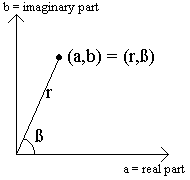COMPLEX:  The '85  has a built in complex number type. In the rectangular form  (a,b)  that represents the complex number a+bi where a and  b are real numbers and i is the imaginary unit whose square is  -1.  Complex numbers can also be represented in polar form as (r,ß) where r is the modulus and ß is the angle.  Complex numbers can be added, multiplied, etc. like real numbers and there are some additional operations: conj gives the conjugate; real gives the real part; imag gives the imaginary part; abs gives the modulus; angle gives the angle.

EX1:   To choose polar coordinate mode,  PolarC, press [2nd] [MORE] to bring up the mode screen, arrow down 4 and over 1 to highlight PolarC and press [ENTER].  Press [EXIT] and enter  (1,1).   Can you explain the result?

EX2:  Redo the first exercise then press [2nd]  to bring up the complex number menu. Press [MORE] [F2] to convert your complex number back to rectangular form.  Yeah?  If you prefer rectangular mode, go back to the mode page and switch back to  RectC before continuing.

EX3:  Find all solutions for the polynomial equation

x4 - 14x3 + 78x2 - 206x + 221 = 0
using the polynomial solver feature. Enter [2nd] [DEL] to bring up the polynomial solver page and enter the coefficients of the polynomial solver as shown. Press [F5] for solve, voila - er, sort of. As you can see in the second illustration here,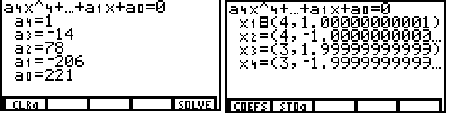the solutions are given in complex form (note these all have non zero imaginary parts) but the solver has a rounding error in the twelfth digit: what should the solutions really be? How can you check this?  Use pencil and paper.

STRING:  A character is what you get by pressing a single key or a key preceded by a [2nd] or an [ALPHA] (sometimes you get more than one character doing this.)  A string is then just a sequence of these characters enclosed by "" marks.  There are a lot of operations you can perform on strings, but you can't evaluate one - it doesn't represent anything but itself. You can store a string to a variable, as in    "ßze5^E"->S so that when you enter S and press [ENTER], the TI-85 returns ßze5^E. You can get the length of a string (the number of characters in the string) using the lngth function and you can get a substing using the sub function.  Strings can be "added" (concatenated) with the "+" operation.  A string with the right syntax can be converted to the EQU data type using the  St>Eq function.

EX1:   Press [ALPHA] twice to lock the TI in ALPHA mode and then type in your name using the blue ALPHA keys - note that pressing ALPHA twice locks in the ALPHA mode.  Press [ENTER]. What's up?

EX2:  Press [2nd]  to bring up the string menu. Press [F1] to get a quotation mark (") - now repeat EX1.

EX3:  Store your name to the variable N  ("<your name>" -> N [ENTER]) then recall your name by entering  N: [ALPHA]  [ENTER].

EX4:  Enter  N+N.  Is it what you expected?  How about  N*N ?

EQUATION: The equation data type is a string that has been parsed by the TI-85's processor for interpretation as a mathematical expression.  This is an innovation of the graphing calculator that sets it apart from its predecessors - but it's fairly elaborate to understand in detail.  An equation in the TI-85 is a string which has been interpreted as a mathematical expression that can be evaluated, solved in the solver or (if there is one independent variable, x) graphed on the graph screen.

LIST: A variable of type list can hold a sequence of real numbers, complex numbers or expressions that can be evaluated as such.  The sequence is delimited by braces { }.  A list can't hold characters (that's what a string is for) nor can it hold strings.

EX1:   Press [2nd] [-] to bring up the list menu.  Enter [F1] [2nd] [^] [,] [2nd] [LN]  [,] [2nd] [LOG]  [F2] [STO>] [TAN] to get "{π,e^1,10^1}D".  Press [ENTER]. You should have:
{3.14159265359 2.71828182846 10}
- to see the whole thing press the right arrow a few times.  We stored this list to D so we could use it later.  Try D+D, D*D, D/D and D^D.  Do these make sense?  How?  Try [2nd] [-] to bring up the list menu and then press [F2] [ALPHA] [TAN] [ENTER] to sort the list.  How did it change?

EX2:   It's easy to create a very long list of numbers in arithmetic or geometric progression using the seq function. Put  2  in x using  [STOå] [x-VAR] [ENTER].   Type [2nd] [X] to bring up the math menu and then [F5] [F3] to get  "seq(" on the homescreen.  Now enter [x-VAR] [^] [ALPHA]  [,] [ALPHA]  [,]  [,]  [,]  [)] [STOå] [SIN] to get seq(x^N,N,1,100,1).  Press [ENTER].  The TI-85 will take awhile to compute the first  100  powers of  2  (currently in x) and store the result in the variable named  "B."  B  is static however - that is, changing what's in x now will not change B.  To see this put  3  in x and recall B - no difference, no?

EX3:  The previous example generated a geometric series using a variable exponent, but any expression whose variables contain values can be used to generate the sequence.  A sequence of 100 pseudo random numbers can be saved to a list by entering

seq(sin(x),x,100,10^4,100)üB.
This is a list of values for sin(x) where x starts from 100 and goes to 10,000 by steps of 100.  Are these numbers really random, or do they follow a pattern?   It is hard to see a pattern using the right arrow to scroll through the 100 12-digit numbers.  To see a graphical representation take the  B  list as the y values and enter a list for the  x  values by storing a list to A:  seq(x,x,1,100,1)üA.   To see the plot, open the graphics screen by pressing [GRAPH]. Set the range with [F2]. Your ranges should look like: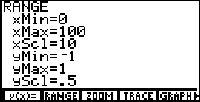Why? Now [EXIT] and make a scatter plot. The fastest way to get "Scatter" is using the catalog. Type [2nd] [CUSTOM]  [ENTER]. This will produce "Scatter" on the home screen. Now enter [ALPHA] [LOG] [,] [ALPHA] [SIN] so you have "Scatter A,B" press [ENTER] and wait for the show. Pressing [CLEAR] now will remove the menu from the screen so you can see the whole plot. Are these points randomly distributed? Why or why not?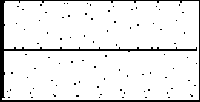VECTOR and MATRIX: A variable of type VECTR is similar in many ways to a list. Like a list, a vector contains a sequence of real or complex numbers or expressions containing real or complex valued variables. In fact you can convert a list to vector or vice versa using the li>vc and vc>li commands. Aside from different delimiters (brackets [] instead of braces {}) The main differences are in what you can do with them. While you can't have a list of lists, you can have a vector of vectors; it's called a matrix. You can sort a list, but not a vector (unless you convert it to a list, sort it, then convert it back); you can multiply lists of the same dimension, but you can't multiply vectors - though you can multiply matrices, as we'll see in the examples to follow.  A vector with 2 or 3 real number elements implicitly represents a point in the plane or in 3-space and can be converted to rectangular, polar, cylindrical or spherical form (if you haven't studied vector calculus, some of these may be unfamiliar.) Matrices are very powerful mathematical objects that have many uses - not least of which, solving systems of linear equations.
EX1:   Type [2nd] [(] to get the bracket for delimiting a vector. Now type in some numbers separated by commas, say  [,]  [,] .  End the vector with [2nd] [)] and store it to A with [STO>] [LOG] [ENTER].  You've got a vector valued variable, A! What can you do with it? You can't multiply it: A*A will give you a data type error; but you can add it to itself: try A+A, just like a list, no? You can also multiply or divide it by a constant. Try 2*A and A/2.
Whoopee. So what's the big deal? Well, this is largely the stuff of Vector Calculus and Linear Algebra - so I won't get into it deeply here. The next exercise illustrates how to use matrices to solve a system of equations, an Intermediate Algebra type problem.
EX2:   Suppose you want to solve the system,
5W + 2X + 3Y + 4Z = 56
4W -  X + 2Y      = 19
X - 5Y + 2Z = -3
W      - 2Y +  Z =  4
using the TI-85. The easiest way is to use the SIMULT page, but suppose your teacher wants you to show row operations one at a time!  Here's how.  Type [2nd]  to bring up the matrix menu. Press [F2] [LOG] [ENTER] to create a matrix named A.  At this stage the screen will display: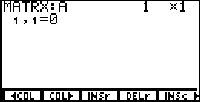In this case, the augmented matrix associated with this system has 4 rows and 5 columns (the fifth column is the right hand sides of the equations) so type  [ENTER]  [ENTER] and the cursor will move down to where you can enter the first row. Type  [ENTER]  [ENTER]  [ENTER]  [ENTER]   [ENTER] and continue on typing in the other rows. Once the matrix is entered type [EXIT] [ALPHA] [LOG] [ENTER] to see the matrix on the homescreen. Does it look ok? Bring up the matrix menu and hit [F4] for the matrix operations menu. [F4] [ALPHA] [LOG] will reduce A to row echelon form. Also [F5] will give you reduced row echelon form, but the teacher wants to see the steps one row operation at a time. For these tools press [MORE] and you'll see rSwap, rAdd, multR and mRAdd. These are the row operations.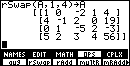Type rSwap(A,1,4)->A to swap the rows 1 and      4.   This is an easy way to get a "1" in the 1st row/1st column position.Now do mRAdd(-4,A,1,2)->A to add -4 times the first row to the second row.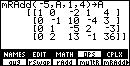To finish off the first column add -5 times the first row to the fourth row with mRAdd(-5,A,1,4)->A.   Ok? First column complete!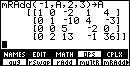Now multiply the second row by -1 to get a 1 in the 2nd row/2nd column position: multR(-1,A,2)->A.  Subtract the second row from the third.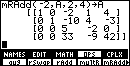Subtract twice the second row from the fourth row.  So far we've been able to avoid fractions, but now we have a bit of a problem. This could be handled in a number of different ways.Divide the third row by 5 (multR(1/5,A,3)->A) and then subtract 33 times the third row from the fourth.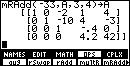Dividing the last row by 4.2 shows that  z=10.  Back-substituting yields the other solutions: y=4, x=-3 and  w=2.

PROGRAM:  Program names are also variable types. A program is a recording of a sequence of commands that you could enter by hand one at a time by hand - only the TI-85 can execute them very quickly!  It can also choose to execute different command sequences depending on this or that condition at one point or another. There is a section with program writing tips later in this document.

By the way, it is worthwhile to take a moment to ponder the difference between a variable and function. A program can be thought of as a function if it accepts input and produces output uniquely determined by the input.  A program can be thought of as a variable - in some sense, they are one and the same beast . Like a function, a variable's value can extend over any predefined range, but it is only a function if its value depends on another value in a well defined way.

EX1:   To define a program type [PRGM] [F2].  Let's write a program to plot one cycle of a function of the form f(x) = D + A*sin(B*(x - c)). The calculator is in ALPHA mode, so type in a name for the program, say "SINE" then hit [ENTER] and you're ready to start entering code. At this stage you would ordinarily follow the program design procedure describe later in this document, but for now, just enter the program as shown: (don't enter the comments)
: Prompt A,B,C,D     //A quick way to get parameter values
: D-AyMin            //Size the graph window
: D+AyMax          // Bring up the range menu with
: CxMin               // [GRAPH] [F2] as a shortcut to xMin, etc.
: C+2*pi/BxMax   // The wavelength is 2*pi/B
: "D+A*sin(B*(x-C))"->S //Create a string to hold the function
: St>Eq(S,y1)                 // Convert the string to an equation
: DrawF y1            // Hit [GRAPH] [MORE] [F2] [F5] for DrawF
Press [EXIT] to save the program in memory. To run the program type [PRGM] [F1] and look for the program name on the menu. What do you think?

In the sense that SINE is a name that holds the code of the program, it's a variable of type PRGM.  In the sense that SINE is a program that takes input and produces output unique to that input, it's a function.

Reserved Variable Names
Some of these variable names are reserved, like "Ans," and can't be written to directly. Entering   1->Ans will cause the '85 to issue a syntax error, for example. Some of the reserved variable names to watch out for are:  Ans, n, a, b, c and corr.

Also be careful with x, y, t, r, equ, exp, Q1...Q9, and QI1...QI9 since the are used by TI-85 when graphing and/or using the solver.

The variables y1...y99, r1...r99, xt1...xt99, yt1...yt99 and Q'1...Q'9 must hold "equations" - that is, variables of type EQU.

"xStat" and "yStat" must be variables of type LIST.

lower and upper must be real numbers, as must xMin, yMax, etc.

Home Screen Display
The Home screen is where you do basic calculations.

You cannot display text on the graph screen, nor graphs on the home screen, so when designing a  program, choose either the more time-consuming good graphics graph path or the easier home screen path.

The TI-85 screen is made up of 127*63=8001 pixels (a pixel is a 'point' on the screen that can be dark=on or light=off.)  These pixels are arranged in a rectangular array 127 pixels wide and 63 pixels high.

Q: Are the pixels themselves square? How can you tell?
A character is a combination of 35 pixels - arranged in a rectangle 5 pixels wide by 7 pixels high. Look very carefully at the characters in "10^" produced by pressing [2nd] [LOG].  If these were enlarged, they might look like:
ž
ž ž
ž žžž ž   ž
ž ž ž
ž ž ž
ž ž ž
ž žžž
There are two 35 bit characters here.  The "10" is crowded into a lower portion of one 5 by 7 spot and the carat into an upper part of the next.  Note that there is a line of pixels separating each row and column of characters so that there are 7 horizontal separators needed, giving a total of 7*8+7=63 rows of pixels.  22 vertical separators are used (there's one on the far left and one on the far right, for a total of 21*5+22=127 columns of pixels.

But these appear on the home screen.  Of course, the same pixels are used for the graph screen.   Pressing [GRAPH] brings up the graph screen and the first 5 of the 13 main graph menu options.

Let's explore the graphics tools available here.

Graphing on the TI-85
The graph screen, accessed by pushing [GRAPH] or executing a DrawF command allows you create, edit, and store graphs and pictures.  There are many types of graphs and many operations that can be performed on them. Let's skim the surface a bit, shall we?
The Graph Screen
Graph Tools, A Quick Overview:

y(x)=    This allows you to insert functions to graph.
RANGE  Allows you to set the x and y ranges of the graph.
ZOOM   As the name implies, this allows you to zoom in and out.
TRACE  Trace the graph of a function displaying the x and y values.
GRAPH  Graphs the current function.
MATH  LOWER  These are used in combination with xMin and xMax and
UPPER  ZOOM  change the range of the graph window and/or some functions.
ROOT   Uses the solver to find zero of the function between LOWER and UPPER.
dy/dx  Compute the slope of a line tangent to the curve at the cursor's x-value.
Sf(x)  Compute numerical integral from LOWER to UPPER.
FMIN   Compute the minimum value of a function between  LOWER and UPPER.
FMAX   Compute the maximum value of a function between  LOWER and UPPER.
YICPT  Compute the y-intercept (f(0)).
ISECT  Find where two functions intersect between   LOWER and UPPER.
DIST   Find the straightline distance between two points on function(s).
ARC    Find the distance along the curve of a function between two points.
TANLN  Draw the line tangent to a curve at a point.
Line   Draws a line from starting point and again to ending point.
Vert   Draws a vertical line from the bottom to the top of the screen.
CIRCL  Draw a circle with radius selected by two points.
DrawF  Draw a function - you must supply a variable of type EQU.
PEN    Turn the pen on and off with ENTER and draw with arrows.
PTON   Turn pixels on by positioning the cursor and pressing ENTER.
PTOFF  This (or PTCHG) will turn pixels off (or change to its opposite.)
CLDRW  Erase the all drawings on the screen - the active functions remain.
CLDRW  Erase the all drawings on the screen - the active functions remain.
TanLn  Draws a line that is tangent to function at the x value.
DrInv  Draws the graphical inverse of the function provided.
FORMT Contains graph formating options, such as show x/y axes.
StGDB Store all the range and format preferences, all functions.
RcGDB Recalls a graph database stored previously.
EVAL  Function evaluated and cursor positioned at given x.
StPic Similar to StGDB but only stores the graph itself.
RcPic Recalls a picture or graph stored previously.

Graphing Examples
EX1:   Where do the graphs of sin(sin(sin(x))) and cos(cos(cos(x))) intersect?  This is awkward if not impossible to solve algebraically - which makes it a good candidate for numerical methods.  Press [GRAPH] [F1] and enter
y1 = sin sin sin (x) and
y2 = cos cos cos (x)
With  xMin=0, xMax=100, yMin=-1 and yMax=1 we have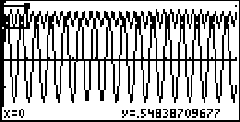Note that we have too many oscillations and there are no points of intersection below the axis, so I've created a zoom box on a portion around the upper left where there may be some intersection. The coordinates of the lower left corner of the zoom box are shown at the bottom of the screen.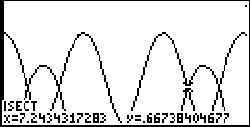After zooming in, you could simply trace and zoom again for accuracy - I pressed [MORE] [F1] [MORE] [F5] to initiate the ISECT function moved the cursor some and pressed [ENTER] twice and '85 came up with the point of intersection shown at left. Notice that the third of four points of intersection in this window is highlighted.

Ex2:     Let's try graphing in polar mode where  r2 = x2 + y2  and θ  is the polar angle.  This is like the situation with complex numbers described previously.  Press [2nd] [MORE] to bring up the mode page and scroll down and right to highlight Pol and press [ENTER].  Now hit [GRAPH] and you'll see r(θ)=  in the [F1] box instead of  y(x)= .

Ok, so instead of entering a function where the vertical coordinate, y, is a function of the horizontal coordinate, x - we have the distance from the origin, r, as a function of the polar angle, θ.  This makes the equations of some curves simpler.  The circle of radius 1/2 centered at (1/2,0) is  y = ±sqrt(x-x2) in rectangular coordinates and r = cosθ  in polar form.  Writing the polar function for the heart shaped (cardioid) function r(θ) = 1 - sinθ  in rectangular forms yields the unwieldy sqrt(x2 + y2) - (x2 + y2) = y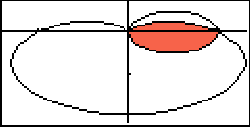What if we want to find the area common to the interiors of these closed curves?  To see this area on you '85, hit the  r(θ)=  key and set  r1=cosθ  and r2=1-sinθ   and then [GRAPH].   With the ranges set for θMin=0 θMax=6.2832, xMin=-1.4, xMax=1.4 we get the graph shown here (color is added to the overlapping region by Photo Shop - the Shade feature only works on the rectangular page.)  Another awkward gap is that you can't measure areas with the integrating function as you can on the rectangular page.

Not to worry! The half circle has area pi/8 (half of pi*r2).  For the cardioid, (r=1-sin(θ)),  integrate r2/2 over θ  in the interval from 0 to pi/2:

pi/2
1/2S(1-2sin*(x)+sin2(θ)) dθ
0

using the identity sin2θ = (1-cos2θ)/2, apply the fundamental theorem of calculus:

pi/2
1/8|(4θ+8cosθ+2θ-sin2θ) dθ  = 1/8(3pi-8)
0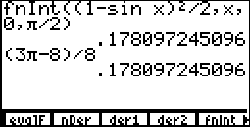Adding in the area of the half circle we have a total area of pi/2 - 1. Here's a screenshot of my check on the '85 at left - just checking that the portion of the area enclosed by the cardioid is right. fnInt is the numerical integrator which is accessible by pressing [2nd] [<division>] to bring up the calculus menu, and then [F5].

Ex3:    To set parametric mode hit [2nd] [MORE] and scroll down and over to highlight Param and then [EXIT].  One of my favorite shapes which is best described using parametric equations is the epitrochoid. The epitrochoid is a shape drawn out by a point on a circle which is rolling around on another circle.  Press [GRAPH] [F1] and type in

xt1 = sin t + (sin (3*t))/4
yt1 = cos t + (cos (3*t))/4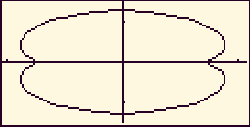and then [2nd] [F5] to see the graph use zoom box or set the range to tMin=0, tMax=6.28, xMin=-1.1, xMax=1.1, yMin=-1.5, yMax=1.5 and you'll see a peanut-shaped graph. This is the shape employed by the rotary engine. To compute various pressures inside the engine, you need to compute the area enclosed by the epitrochoid. It is extremely difficult (if not impossible) to express this shape in rectangular or polar form - fortunately, we don't need to. To compute the area in the first quadrant, simply integrate       Sxdy = Sx(t)(dy/dt)dt =

0
= S(sint+(sin(3t))/4)(-sint-3sin(3t)/4)dt
pi/2

pi/2
= S(sin2t+sintsin(3t)+3sin2(3t)/16)dt
0

This can be evaluated symbolically without much trouble, but let's use the numerical integrator on the '85. Bring up the CALC menu, hit [F5] and type in

fnInt((sin x)2+sin x*sin (3*x)+3*(sin (3*x))2/16,x,0,pi/2)

which evaluates to 0.93266 and thus we have a total area 4 times that: 3.73 square units.

EX4:     The fourth and final type of graph is the numerical differential equation solver/plotter. Type in [2nd] [MORE] and scroll down and over to highlight DifEq and press [EXIT].  Now when you press [GRAPH] the [F1] key activates Q'(t)= where you can enter in a system of first order differential equations.

A lorenz system is described by the solution of three simultaneous differential equations:

• dx/dt = -s(y-x)
• dy/dt = rx-y-xz
• dx/dt = -dz+xy
The phase plot of x versus z is plotted for s = 10, r = 28, and d = 8/3 yielding the beautiful (and now well known) Lorenz Attractor.  On the '85 we use Q1, Q2 and Q3 in place of x, y, z. We can use  s, r, c as parameters if we initialize them from the homescreen: 10->s, 28->r and 8/3->d.  Now, back in the Q'(t)= window enter
Q'1=s*(Q2-Q1)
Q'2=r*Q1-Q2-Q1*Q2
Q'3=-d*Q3+Q1*Q2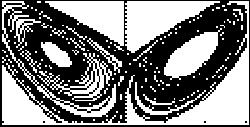From the [GRAPH] menu push [F3] to set the initial conditions as say, QI1=-10; QI2=-10; QI3=30 and from [F2] set the range as tMin=0; tMax=50; tStep=.01; tPlot=0; xMin=-15; xMax=15; yMin=10; yMax=40. Also with [F4] choose axes: x=Q1; y=Q3. Finally, hit [F5] to graph and kick back for about 30 minutes.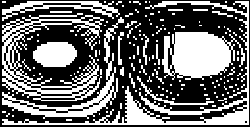Here's a picture of the same system with the same initial conditions, only viewing the plot of x=Q2; y=Q3. From these two projections you can imagine what the 3-dimensional graph might look like. To see a picture of it and read some more about how Lorenz used this system to model air flow inside a heated box, check out this site: http://www.students.uiuc.edu./~ag-ho/chaos/lorenz.html

Programming
Why program the TI-85?

Let me offer some reasons:

1.  The '85 is extremely small, lightweight and battery operated.
It can follow you almost anywhere.
2.   It is a very versatile machine. You can write anything from
operating systems to accounting programs, games to programs
3.  The programming skills needed for the TI-85 carry over to
larger, more complicated platforms.

Disp, DispG, PrtScrn
The  Disp  function writes data on the screen below any data already on the screen, with the first letter all the way to the left if it is displaying letters, and the last digit all the way to the right of the screen if it is displaying a value. The  Disp  function has the following forms:
:Disp <variable>      for a string or a value
:Disp <"string ">      for a string
:Disp  by itself displays the home screen.   The  Disp  function can also display several strings separated by a comma:
:Disp "The residue is:", N, "mod", M
If  N=1  and  M=89,  this would display
The residue is: 1 mod 89
The DispG function switches to the graph screen and displays the current graph.  The PrtScrn function is not a common one -- it prints the screen to a Macintosh or PC printer connected to it with the TI-85 Graph link. Without the link it acts like Pause. Put ClLCD and PrtScrn on a line by itself.

Input, Outpt and For Loops
Input/output commands are used to make a program communicate with the user - that's you, sir - er, or, Ma'am.

The Outpt()function is used to make the program print something on the screen. The general form is:

:Outpt(<row>, <column>, <argument>)
The TI-85's screen is 8 characters high and 21 characters wide.  If you wanted to print "MIDDLE" in the middle of the screen, issue the command
:Outpt(4,7,"MIDDLE")
or, in general choose the column number = 11 - int(number of characters/2) to center a word. The argument is what is to be printed. If you use quotation marks, the TI-85 will print exactly whatever is in the quotation marks - if you don’t, it will print the value of the variable with that name. For example, say there is a variable called NAM that contains the words "Hi Jake!" Then
:Outpt(4,8,NAM)
prints   Hi Jake!  on the fourth line.

The "For" loop with syntax

For(x, a, b, s)
<commands executed in "For" loop>
End
repeats a list of commands as x counts from a to b by a step size s.  The default s is 1 - that is, For(N, 1, 10) will execute 10 loops as N counts 1, 2,..., 10.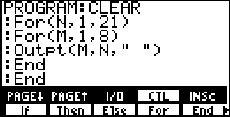EX1:       You can erase a value off the screen without clearing other values by using Outpt to put blank space(s) at that location. See how this technique can be combined with nested "for" loops to clear the screen.  Press [PRGM] [F2] to bring up program edit and then type CLEAR (using the blue keys) then use the [I/O] and [CTL] menus to type in the code shown on the screen shot at right. [EXIT] twice to save the program.  Load up the home screen with some flotsam and jetsam, press [PRGM] [F1] to bring up the program names menu and hunt for "CLEAR" (using [MORE] if necessary) and peck it.  It's a nice illustration of how nested "for" loops work.

As such, it's worth pausing to examine further.  Here's some fought Thor food:

1. How many Outpt commands are issued?
2. How long does the program take to run?
3. Estimate the time per Outpt command.
4. How could you impove this estimate?

5. Try replacing the blank character with something else.
What happens when you reverse the "for" loops?
Of course there's a much faster way to clear the screen: the ClLCD command.

To have the program first prompt you, sir (or madam,) for a character to fill the screen with, write a program called FLLR with the following code:

: Input "Enter a char:",C
: For(M,1,24,3)
: For(N,1,105,5)
: 1+mod(N,21)->H
: 1+mod(M,8)->V
: Outpt(V,H,C)
: End
: End
The character entered can be a string, number or variable. Be sure to use quotation marks to delimit a string ([2nd]  brings up the string menu.)  Note that mod(N,21) gives the remainder when N is divided by 21. Can you explain what happens when you run this program?

The InpSt function is used whenever you want the user to input a string of letters: :InpSt <variable>.   Try substituting  InpSt for Input in this program.  What do you think?

Ex2:     How about taking a string and waving it around on the home screen?  Here's a more complicated program which illustrates a few tricks.

:ClLCD    // Clear the screen
:Degree   // Set degree mode (for sine function below)
:Outpt(4,4,"Enter a string.") //Prompt for input
:InpSt S  //Input a string into variable S
:ClLCD    // Clear the screen again
:lngth S->N // Measure the length of S and store in N
:N->dimL L  // L and T will hold coordinates of
:N->dimL T  // characters on the screen.
:For(K,1,N) // Initialize position arrays
:4->L(K)    // Initial position in middle of screen
:(4,4)->T(K) // T is the old position - complex format
:End         // is used to keep coordinates as real and imag
:1->A     // Is the degree measure of an angle
:While 1  // Loop forever - or until [ON] interrupts
:For(K,1,N) // Write the string
:Outpt(L(K),K+int ((21-N)/2),sub(S,K,1)) // Note horiz. center
:(L(K),K+int ((21-N)/2))->T(K) // Keep track of old pos.
:A+5->A  //Increment angle to simulate wave motion
:int (5+4*sin (A))->L(K) // New vertical position
:Outpt(real T(1+mod(K+1,N)),imag T(1+mod(K+1,N))," ") //erase
:End // End For loop
:End // End While loop
Try substituting  sin (2*A) for  sin (A) or tweaking  other parts of the program. Note that it runs pretty slowly - can it be sped up?
getKy and While
The getKy function checks to see if the user has pushed any keys. If the user has not, it puts a 0 (zero) into variable.   If a key is pressed, then getKy puts a value into variable, depending on which key was pressed. The general form is:
getKy <variable>

Here's a list of keys and their return values:

[F1]-[F5]:                11-15
[2nd] - [<right arrow>]   21-26
[ALPHA] - [<down arrow>]  31-34
[GRAPH] - [CLEAR]         41-45
[LOG] - [^]               51-55
...and so forth, except that [ON] is not numbered, so there is no 101.

Using  getKy with the control command,  While,  is an effective way to branch a program from user input.  Here's a little program fragment to illustrate this:

:0->M
:While M==0
:getKy->M
:End
:Outpt(4,10,M)
The first command puts 0 in a variable called M.   The While command then executes the following command(s) (until End) which, in this case, is the single command to put the value of whatever key is pressed into M.  If no key is pressed, M is 0 and the while-loop continues.  As soon as key is pressed, M takes the value of that key and the loop is exited. At this point, the output command will execute. Try it! Use [2nd]  to bring up the test menu where "==" lives.

Note the relational operator, "==," should not be confused with the assignment operator, "=," which stores an expression in a variable of type EQU. The "equals?" operator, ==, checks to see if two values or strings match, and returns 1 if they match or 0 if they don't match.

The If-Then-Else Controls

The If function makes decisions in a program based on whether or not its argument equals zero.  If the argument equals anything but zero, it does whatever is on the next line after If. The general form is:
:If <argument>
:<stuff done if argument does not equal zero>
:<stuff done in either case>
For example,
:If x==3 //x==3 evaluates to either one or zero
:Outpt(2,1,"Hi Jake")
:DispG
will output "Hi Jake" only if x holds the value 3. In any case, the graph screen is displayed immediately afterwards, so you get a chance to see "Hi Jake"!

The word Then is used after If when you need more than just one statement evaluated after If.  The word End signals the end of the list of things to do. This has the form:

:If <argument>
:Then
:<Things to do if argument does not equal zero>
: . . .
:<Things to do if argument does not equal zero>
:End
:<Stuff done if If condition is false>
The word Else is used to tell the program what to do if argument does equal zero. It must be used with Then.  It is used after the list of things to do if true (not equal to zero) and has an End following it. The form is:
:If <argument>
:Then
:<Commands to do if true (argument does not equal zero)>
:<Commands to do if true (argument does not equal zero)>
:Else
:<Commands to do if false (argument equals zero)>
:<Commands to do if false (argument equals zero)>
:End

Before we go further, let's take a short course in how to design code:
The Design Process
The design process is might be outlined something like this:

1)   Decide what you want your program to do.
2)   Write pseudocode; that is, outline in broad strokes how the flow will go.
3)   Draw graphics, if necessary, on graph paper - images that the program will use.
4)   Work out methods to do difficult routines, drawing on innovation and experience.
5)   Type it in. It's easier to use a computer keyboard, if you have the Link utility.
6)   Test.
7)   Debug.
8)  Add on new features you think would enhance the performance.
9)  Test
10) Debug
11) Make documentation, bundle, other things needed for full program.
12) Release beta version.

Ideally, if you can conceive it (step 1,) you can write it - except that on the TI you are limited by space, execution speed, and deficiencies in the TI language.  Still, it takes considerably more creativity and imagination to dream up a program than to write it.

Step 2 refers to writing on paper, code which uses terms from the language but is just an outline of what will happen.

Step 3 is necessary if you want to test parts of the program before it is completed.  If the Pics aren't finished it won't work.

Step 4 is where software engineers define their skill.  You often run into routines you have no idea how to do.  Use pencil and paper so you can see the whole thing.  Make a flow chart, thread through the commands one at a time, making a list of what variable has what value when.  Think of alternatives, look in the CATALOG for commands that may be useful.

Debugging (aka error checking or troubleshooting) in step 6 is really an extension of step 4.  This is the "check" part of the "guess and check" method.  Sometimes a bug is so elusive, you cannot find it. This is why professional software companies release "beta" versions of their software for free. If enough people try to use the software in crazy ways, its weaknesses usually surface fairly quickly.  Go through the code step-by-step keeping a table of the values of all the variables involved at those points, and do the commands just as the TI would. This may help you to see where, when, and under what circumstances you run into the problem. Try using different input values: good software is idiot proof and will disallow crazy input. By the time you've done all that, you may have new ideas, don't be afraid to start from scratch again.

EX3:  Suppose we dream up an idea to have a character bouncing around the screen. Recall the program fragment using getKy and While to get a pressed key's value and output it:
:0->M
:While M==0
:getKy->M
:End
:Outpt(4,10,M)
The program will, as I imagine it, place an alphabetic character associated with the key in a random position on the screen and set it in motion down and to the right until it hits a "wall," at which point it will "bounce" and continue bouncing until another alphabet key is pressed at which time the new letter will be randomly placed and start bouncing.  To stop the process the [(-)] key will be used.

So that's step 1 in the design process. The pseudo code now might look like this:

Initialize screen and variables
Use the While/getKy technique to wait for and take input
If the key doesn't have an alphabet character, ignore it.
If the key has an alphabet character, place it on a random spot on the screen.
While no new key is pressed, and the character is not at a wall, erase it and
redraw it one spot down and to the right.
Continue this erase/draw procedure until you get to a wall, then bounce.
Yeah?  Now since there are now graphics to draw, we proceed to step 4.  I prefer using the computer to type in the program pieces as I think of them, but paper is preferable to trying to type in stuff on the calculator directly.

Rather than start at the beginning and develop code linearly, it may be better to start in the middle and work outwards.  Can you identify loops that will be employed?  We'll need one loop to accept a character and another to bounce it around once we have it.

Some languages have a "case" statement which can be used to branch on a key press, on the TI, the If-Then-End commands can serve the purpose - but since there is no methodical way to converting the number value to an alphabetic character that I can think of, I think I'll need a separate If-Then-End statement for each key.

Does this mean retyping the code for moving the character inside each Then-End block?  Fortunately, no.  We can write what's called a "subroutine" as a separate program which can be called by the main program.  Let's see how this might work.

Given the alphabetic character, the subroutine will need to to choose its initial position and send it moving with erase/redraw.  It will also have to know when a new key is pressed.  Finally, it will need a way of moving the figure.  Assuming the alphabetic character has already been stored in the variable, S, after some fumbling, I come up with:

:1+int (20*rand)->H   // Choose a random integer between 1 and 21
:1+int (7*rand)->V   // Choose another between 1 and 8 (inclusive)
:1->I   // These are the increment variables, initially set to
:1->J  // move the character down and to the right.
:0->M // M will hold the value of any key that is pressed.
:While M==0   // If a key is pressed, we'll exit the subroutine
:if H==21        // If at the right wall,
:-1->I          // then reverse the horizontal increment (bounce)
:if H==1       // If at the left wall,
:1->I          // bounce right.
:if V==8     // If at the bottom,
:-1->J      // bounce up.
:if V==1   // If at the top,
:1->J     // bounce down.
:Outpt(V,H," ")   // Before incrementing the indices, erase.
:H+I->H          // Increment horizontal position.
:V+J->V         // Increment vertical position.
:Outpt(V,H,S)  // Redraw the character at the new position
:For (K,1,30) // Kill some time (if it moves too fast, you can't see it.)
:1->N        // Whatever...
:End        // End For loop
:getKy->M   // Check to see if a key has been pressed
:End       // End while loop
:Return   // Go back to the main routine.

Enter this code under the name POSSUB.

Now all we need is a routine which will see which key has been pressed and convert its value to an alphabetic character.  The following gets the job done, even if it looks a bit clunky:

:ClLCD
:1+int (20*rand)->H // Initialize the horizontal position.
:1+int (7*rand)->V // Initialize the vertical position.
:0->next  // When next is 1, we exit.
:ClLCD   // Clear the deck.
:0->M
:While (M==0) // Wait for the first key press to get going.
:getKy->M
:End
:Repeat next       // This is the outer loop of the main routine.
:Outpt(1,1,"HEY")  // This was originally for error checking - I left it in.
:Outpt(3,1,M)
:If M==51      // If the value of the key pressed is 51
:Then
:"A"->S       // The "A" key was pressed so load S with "A".
:POSSUB       // Do subroutine stuff with "A" in S.
:End
:If M==52   // Same as above, only the "B" key was pressed.
:Then
:"B"->S
:POSSUB
:End
....         // Repeat If-Then-End for each character of the alphabet.
:If M==103
:Then
:"Z"üS
:POSZ
:End
:If M==105  // If the [ENTER] key is pressed
:Then
:1->next  // Load next with 1 so as to exit the Repeat loop.
:End
:End   // End Repeat loop.

The Repeat function does a series of commands, then checks if a condition is true (does not equal zero). It differs from While in that the <Things to do again if condition is true> get done at least one time, regardless of whether or not the condition is true. Repeat will also create an infinite loop without help if condition is true initially. The While and Repeat functions are just For() loops that don't increment (increase by a value every time around) conditions themselves.

The IS>() and DS<() Commands

The IS<() (increment and skip) command increments the variable by one and skips the next command if variable is greater than <value> . This function has the form:
:IS>(<variable>, <value>)
:<Command to skip if variable exceeds value>
The DS>() (decrement and skip) command decreases the variable by one and skips the next command if the variable is less than <value> . This function has the form:
:DS<(<variable>, <value>)
:<Command to skip if variable is less than value>
The Lbl and Goto Commands
The Lbl and Goto functions allow the program to go to a certain part of the program that has been Lbled. For example,
:<commands>
:Lbl <labelname> ...to here.
:<commands>
:Goto <labelname> Control goes from here...
:<commands>

The Menu() command creates a menu at the bottom of the screen. It has the following form:
where up to 5 positions/string/label combinations can be specified at once.   When the user pushes an F-button, control is passed to the associated <label> .   Only five characters of <"string"> are shown on a tab.

Here's a program using a menu:

:Lbl M1
:Lbl XIS    // [F1] was pressed
:Disp "x is" x
:Goto M1    // Go back to menu
:Lbl GRPH   // [F4] was pressed
:DispG
:Goto M1  // Go back to menu
:Lbl BYE   // [F5] was pressed
:Stop
Can you see how the flow passes back and forth from the menu and the code via  Goto and Lbl commands?

Getting Maximum Graphics on the '85.

Say you're writing a game which involves a bouncing ball.  You could use Outpt(x,y,"o ") to make the ball and form a paddle with \.   This is easy, but higher quality images require using the graph screen. Using the PtOn (X, Y) command, a program can draw an image point by point.  To create graphics point by point is extremely time consuming at the production stage, but it is the secret to the beautiful graphics.

Using the Graph Screen for Animation from a Program

We've already seen animation on the home screen.  Animation on the graphics screen is just as easy, but much more powerful.  Instead of drawing a character at a time, we achieve much higher resolution by drawing a pixel at a time, and much more flexibility by drawing from a library of pre-drawn pictures.  Image drawing algorithms can be relative to a variable point, so the same subroutine can be used to draw a "pic" anywhere on the screen.  Each PtOn command takes some time to execute (though, if the PtOn's are chained together, the total time is not a simple multiple of the the time to perform a single PtOn) so the game is to get the job done with a minimum of commands - this allows you more control over the speed the program runs.  That is, you can always slow it down by inserting dummy commands, but speeding up is _not_ always possible.

Using Line() and  PtOn() in cases where the pic will only require less than, say, 50 pixels is preferable to using ClDrw or RcPic which effect all 127*63 = 8001 pixels.  In cases where more pixels are present, StPic does a better job at keeping things small. And, don't ever forget to set xMax at 127, yMax at 63, and xMin,yMin at 0.

The Graph Tools

The Line() function draws a line between (x1,y1) and (x2,y2).    Line() requires less time than PtOn() if more than one point needs to be turned on - and Line() takes up less memory than several PtOn()s.   The syntax for Line() is:
:Line(x1,y1,x2,y2)
The PtOn(x,y) function "turns on" (darkens) the pixel at (x,y).  Avoid PtOn() in cases where Line() will do. The PtOff(x,y) function is like PtOn, but it makes pixels light (off).

Since the Outpt() command doesn't write to the graph screen, to print text on the graph screen requires drawing the character using the Line() and PtOn() commands.

Make a grid (or use graph paper) the size of your desired letter or number.  Write  y,y+1,y+2,... in a column on the left side of the grid, and x,x+1,x+2,... along the bottom. Then, fill in the boxes (corresponding to pixels) to draw the letter. For example:

y+5   ž
y+4  ž ž
y+3 ž   ž
y+2 žžžžž
y+1 ž   ž
y ž   ž
xxxxx
++++
1234
A short program (say, DrA) to draw this letter could be:
:1->xMin     // Set the graph window so that
:1->yMin     // each integral value of x and y
:63->yMax    // corresponds to a unique
:127->xMax   // pixel.
:FnOff       // Unselect all functions.
:Prompt x,y  // Quick easy way to get input for position
:Line(x,y,x,y+3)
:PtOn(x+1,y+4)
:Line(x+2,y+5,x+4,y+3)     // Draw "A" on the graph
:Line(x+4,y+2,x+4,y)       // screen.
:Line(x+1,y+2,x+3,y+2)
Note that it's dangerous to use "x" here since this is a variable the '85 writes to often. Hey, we're learning - live dangerously!  Just watch out later on!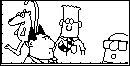When designing a full-screen graphic to be saved as a Pic, make a 127 x 63 grid.  If you have access to a computer graphics software like Photo Shop Pro or Paint for Windows, make a 127 x 63 rectangle and use the software tools available to draw the picture, then transfer to graph paper with a pencil. Then on the TI use the [PEN] tool under the [GRAPH] [MORE] [DRAW] [MORE] menu.   If your range is set as in the DrA program above, you will see the exact coordinates of the pixel the cross hair is on at the bottom of the screen. Maybe this is how the Dilbert graphic shown here was made? I don't who did it, or where I got it. Below is the Photo Shop prototype of Cyclops with Birds I created, together with the TI Pic I made from it: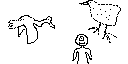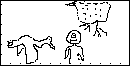Note that I got confused by the coordinate system of Photo Shop (which has y increasing as you go down) so the first bird is too low.  Also, I ad-libbed a bit in the translation.  Just impatient, I guess.

Better yet, in many ways, is the 86iview program available for free at the TI calculator archives:  http://www.ti.com/calc/docs/arch.htm.  Using 86iview I drew the following picture demonstrating the 3-colorability of the trefoil knot, in 2 minutes: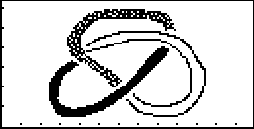Execution Times (times are in thousandths of a second.)
For Loop_______________________________5.50
PtOn__________________________________18.89
PtOff_________________________________16.55
10 PtOns______________________________25.63
10 PtOffs_____________________________25.62
Line (1,1 to 1,1)_____________________28.89
Line (1,1 to 127,63)__________________52.67
Chain of PtOns from 1,1 to 127,63___3255.01